# PSAT Math : Percentage

## Example Questions

### Example Question #2031 : Psat Mathematics

What is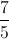as a percentage?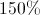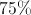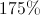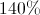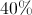Explanation:

Convert the improper fractionto the mixed numeral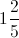or as the decimal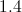.as a percentage is### Example Question #11 : Fractions And Percentage

A family with 6 children, aged 4, 4, 5, 7, 12, and 13 are moving to a new home. They all want the same bedroom, so the parents have a lottery. Each child places their name in once for every year of age (the four year olds each put their name in 4 times, the seven year old puts his name in 7 times, etc.) What is the probability of the chosen child being 4 years old?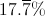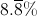None of the available answers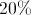It is most likely that the chosen child will be the oldest child.Explanation:

First, we will determine the total number of ballots: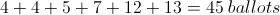Since there are two four year olds, and this question is asking the probability of the chosen child being four, the probability is: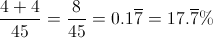### Example Question #1 : How To Find Percentage From A Fraction

Three hens each lay 20 eggs for a farmer. Two-fifths of the first hen's eggs are brown. One-fourth of the second hen's eggs are brown. Seven-tenths of the third hen's eggs are white. What percentage of all the eggs are brown?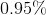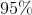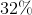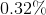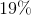Explanation:

The first hen lays 8 brown eggs: 2/5 * 20 = 8

The second lays 5 brown eggs: 1/4 * 20 = 5

The third lays 6 brown eggs: 3/10 * 20 = 6

Add up the brown eggs and divide by 60, the total number of eggs:

19/60 = 0.31666667 = 31.67%, or 32%.

### Example Question #2 : Fractions And Percentage

There is a sale in a store and every item is discounted 23%. If a shirt is normally $18.59, what is the price of it after the discount? Possible Answers: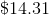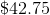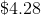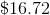Correct answer:Explanation: We can right 23% as a fraction and set that equal toover the original price.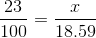From here we cross multiply and divide.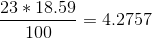We round this number to$4.28. This is the amount of discoount we need to apply to the original price. To do this we subtract 4.28 from 18.59.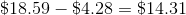### Example Question #2 : Fractions And Percentage

Louisa and Caroline are trying to save up to start a small business together. Louisa has saved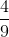of their goal money, and Carolina has saved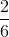of their goal money. About how much of their goal money have they saved up together?

18%

78%

56%

64%

45%

78%

Explanation:

The goal of this problem is to solve for how much Louisa and Caroline have saved, together, towards their goal money for starting a small business.  In order to do that, you must add what Louisa has saved to what Caroline has saved, then convert the fraction into a percentage.

Add Louisa and Caroline: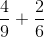Find the lowest common multiple for the denominator: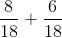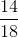Simplify: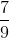To convert a fraction into a percentage, simply multiply the numerator by 100 and divide by the denominator. There are other methods, but this is the easiest.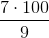Equals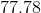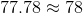### Example Question #71 : Percentage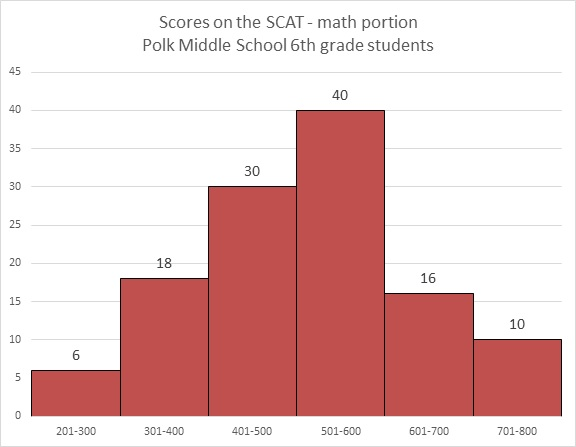Refer to the above bar graph.

What percent of the students achieved a score 500 or below?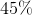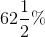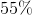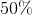Explanation:

6 students achieved a score of 201-300; 18 achieved a score of 301-400; 30 achieved a score of 401-500. Add these: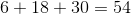The number of students who took the test is the sum of the students who finished in the six ranges: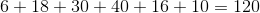The question is now to find out what percent 54 is of 120, which can be calculated as follows: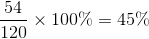### Example Question #1 : How To Find The Whole From The Part With Percentage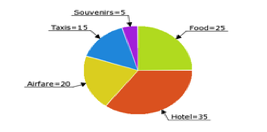David's trip expenses are pictured in the above pie chart (numbers = % of his total expenses). If he spent $75 on taxis, how much did he spend on hotel and souvenirs combined? Possible Answers:$225

$40$200

$175$250

$200 Explanation: David spent$75 on taxis, which were 15% of his total expenses on the trip. He therefore spent 75(100/15) = $500 on the trip altogether. The hotel and souvenirs make up 35% + 5% = 40% of his total expenses. 40% of 500 is$200.

### Example Question #2 : How To Find The Whole From The Part With Percentage

30% of what number is 20?

Solve to the nearest hundredth.

None of the other answers

66.67

1.5

150%

0.67

66.67

Explanation:

This is a very basic form percentage question. This can be rewritten:

0.3 * x = 20

(Remember, the word "of" in a word problem indicates multiplication, while the word "is" indicates an equals sign).

Solve for x: x = 20 / 0.3 = 66.67

A toy is on sale for 43% off. Its sale price is $21.37. What is the full price? Possible Answers: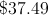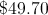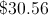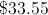None of the available answers Correct answer:Explanation: An algebraic expression for this item is: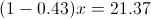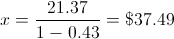### Example Question #1 : How To Find The Whole From The Part With Percentage Twenty-six students planned to contribute an equal amount to purchase a gift for their teacher. After 18 students had paid, they had collected$76.50. What is the total price of the gift?If $76.50 had been collected after 18 students had paid, we can determine how much each student contributed:$76.50/18 = $4.25 per student Now we can multiply this by the total number of students (26) to get the full price of the gift: 26 x$4.25 = \$110.50